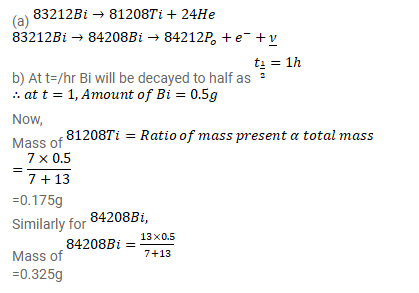# Can disintegrate either by emitting an ????-particle or by emitting a 83212Bi-particle.

Question:

Can disintegrate either by emitting an $\alpha$-particle or by emitting a 83212Bi-particle. (a) Write the two equations showing the products of the decays. (b) The probabilities of disintegration by $\alpha$-and $\beta$-decays are in ratio $7 / 13$.

The overall half-life of ${ }^{212} \mathrm{Bi}$ is one hour. If $1 \mathrm{~g}$ of pure ${ }^{212} \mathrm{Bi}$ is taken at $12: 00$ noon, what will be the composition of this sample at 1 p.m. the same day?

Solution: